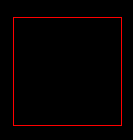# FlatRedBall.Math.Geometry.Polygon.IsPointInside

## Introduction

The IsPointInside method returns whether an absolute X/Y position is inside the calling Polygon. This function takes 3D position objects (such as Vector3) but ignores the Z value. Only X and Y are considered.

## Code Example

The following code creates a Polygon that is in the shape of a rectangle. When the user moves the cursor in the polygon it turns red; otherwise it is yellow.

```using Microsoft.Xna.Framework.Graphics;
using FlatRedBall.Math.Geometry;
using FlatRedBall.Input;
```

Define the Polygon at class scope:

```Polygon polygon;
```

Add the following in Initializing after initializing FlatRedBall (or CustomInitialize if using Screens):

```polygon = Polygon.CreateRectangle(3, 3);
IsMouseVisible = true;
```

Add the following in Update (or CustomActivity if using Screens):

```if (polygon.IsPointInside(
InputManager.Mouse.WorldXAt(polygon.Z),
InputManager.Mouse.WorldYAt(polygon.Z)))
{
polygon.Color = Color.Red;
}
else
{
polygon.Color = Color.Yellow;
}
```## IsPointInside and ForceUpdateDependencies

The IsPointInside method requires that the Polygon has constructed its internal vertices. This means that you must either:

If neither of the above has happened, then the internal vertices will not be set and this function will always return false.